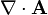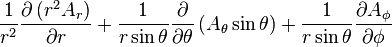# Divergence of newton's law of gravitation

#### bitrex

I am studying vector calculus, and I saw the following result in a physics text:

$$g = -\frac{m}{r^3}\vec{r}$$
$$r^2 = x^2 + y^2 + z^2$$
$$\vec{r} = ix + jy +kz$$

$$\nabla \cdot g = 0$$

I'm not sure how this was done. Is the product rule used somehow? What happened to the extra power of r? Thanks for any advice.

#### Jolb

It helps to use the spherical version of divergence.=I don't expect you to know the spherical version (few people know it by heart), but doing that problem in cartesian coordinates is only for masochists.

This is actually an interesting problem, though. That field is the gravitational field of a point mass (or electric field of a point charge) at the origin. Since there is a nonzero field everywhere, you'd expect there to some source of the field, i.e., a point of nonzero divergence!

You'll find that if you plug your field into that formula for the divergence, it is zero everywhere but has a value of 0/0 at the origin. In many texts, they give an argument for why that field's divergence should actually be a dirac delta function (i.e., there is an infinitely small region of nonzero, finite divergence at the origin.)

Last edited by a moderator:

#### coki2000

Okey.But why don't you use the cartesian coordinates, why the spherical coordinates??and why masochism.

Last edited by a moderator:

#### D H

Staff Emeritus
Computing $\nabla\cdot \vec g$ in spherical coordinates is easy. In spherical coordinates, the gravitational acceleration is

$$\vec g= -\frac {mG}{r^2}\hat r$$

Thus with the caveat that $r\ne 0$,

$$\nabla \cdot \vec g = \frac 1 {r^2} \frac {\partial}{\partial r}\left(r^2\left(-\frac {mG}{r^2}\right)\right) = \frac 1 {r^2} \frac {\partial }{\partial r}\left(-mG\right) = 0$$

The masochism arises in computing it in cartesian coordinates. Try it.

#### coki2000

Computing $\nabla\cdot \vec g$ in spherical coordinates is easy. In spherical coordinates, the gravitational acceleration is

$$\vec g= -\frac {mG}{r^2}\hat r$$

Thus with the caveat that $r\ne 0$,

$$\nabla \cdot \vec g = \frac 1 {r^2} \frac {\partial}{\partial r}\left(r^2\left(-\frac {mG}{r^2}\right)\right) = \frac 1 {r^2} \frac {\partial }{\partial r}\left(-mG\right) = 0$$

The masochism arises in computing it in cartesian coordinates. Try it.
But the divergence of gravitational acceleration could be $$-4\pi.GM$$ isn't it.

#### D H

Staff Emeritus
But the divergence of gravitational acceleration could be $$-4\pi.GM$$ isn't it.
You are thinking of the integral of the divergence over some volume,

$$\iiint_V \nabla \cdot \vec g \, dV = \oint_S \vec g \cdot d\vec S = -4\pi mG$$

This is why some write the divergence as a (three dimensional) delta function.

#### coki2000

You are thinking of the integral of the divergence over some volume,

$$\int_V \nabla \cdot \vec g \, dV = \oint_S \vec g \cdot d\vec S = -4\pi mG$$

This is why some write the divergence as a (three dimensional) delta function.
Thanks.Alright what is its proof that $$\int_V \nabla \cdot \vec g \, dV = \oint_S \vec g \cdot d\vec S = -4\pi mG$$? Do you explain to me?

#### D H

Staff Emeritus
The first equality is the divergence theorem. The second equality results from evaluating the surface integral.

### The Physics Forums Way

We Value Quality
• Topics based on mainstream science
• Proper English grammar and spelling
We Value Civility
• Positive and compassionate attitudes
• Patience while debating
We Value Productivity
• Disciplined to remain on-topic
• Recognition of own weaknesses
• Solo and co-op problem solving